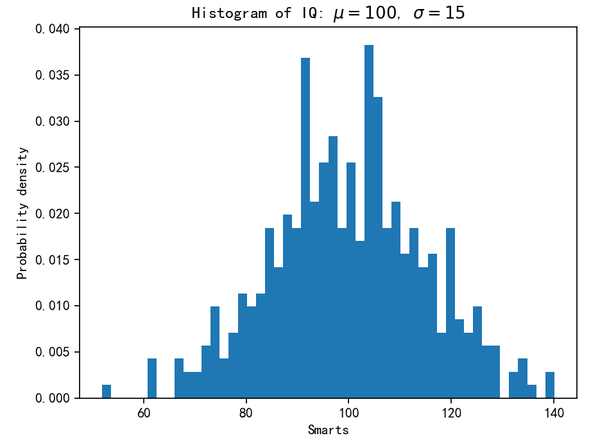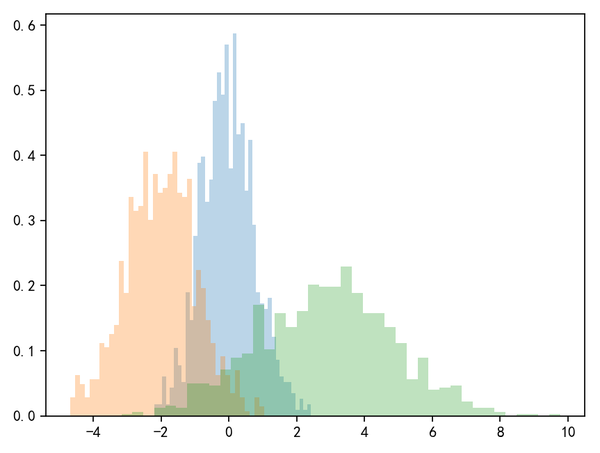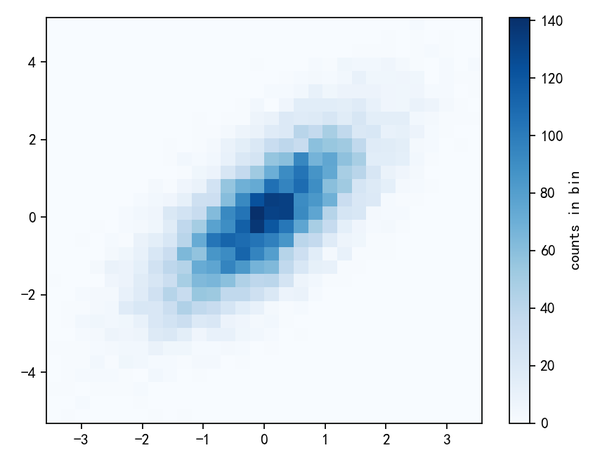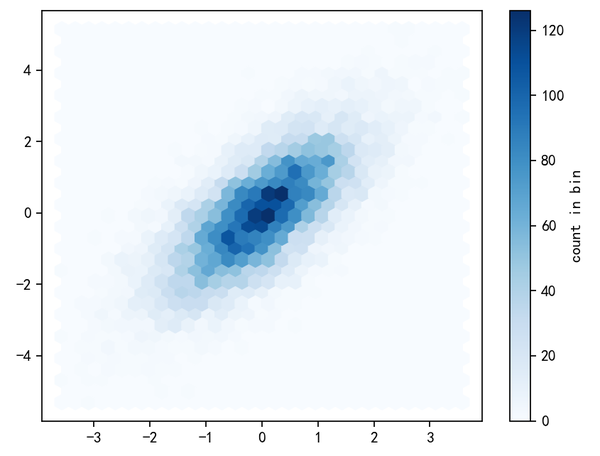# 直方图

np.random.seed(2019)

# 创建数据
mu = 100  # 分布的均值
sigma = 15  # 分布标准差
x = mu + sigma * np.random.randn(400) # 生成400个数据

num_bins = 50  # 分50组

plt.hist(x, num_bins, density=1, )

plt.xlabel('Smarts')
plt.ylabel('Probability density')
plt.title(r'Histogram of IQ: $\mu=100$, $\sigma=15$')plt.hist(np.random.randn(1000), bins=30,normed=True, alpha=0.5, histtype='stepfilled', color='steelblue', edgecolor='none')


histtype直方图的类型，可以是'bar'、 'barstacked'、'step'和'stepfilled'。

x1 = np.random.normal(0,0.8,1000)
x2 = np.random.normal(-2,1,1000)
x3 = np.random.normal(3,2,1000)

params = dict(histtype='stepfilled', alpha=0.3, normed=True,bins=40)

plt.hist(x1, **params)  # 以字典的形式提供参数
plt.hist(x2, **params)  # 在同一个子图中绘制，颜色会自动变化
plt.hist(x3, **params)mean = [0,0]  # 忽略数据的创建过程
cov = [[1,1],[1,2]]
x,y = np.random.multivariate_normal(mean, cov,10000).T

plt.hist2d(x,y,bins=30,cmap='Blues')  #以蓝色为基调
cb = plt.colorbar()  # 插入颜色条
cb.set_label('counts in bin')  # 设置颜色条的标签hist2d是使用坐标轴正交的方块分割区域，还有一种常用的方式是正六边形也就是蜂窝形状的分割。Matplotlib提供的plt.hexbin就是满足这个需求的：

plt.hexbin(x,y,gridsize=30, cmap='Blues')
plt.colorbar(label='count in bin')#### 评论总数： 1plt.hist2d函数的前两个参数可以是一维数组，不能是多维;plt.hexbin函数前两个参数可以是一维数组也可以是多维。